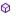Press on the image to return to the main documentation page.

# EQlib

EQlib

### EQlib

#### Permissions:

android.permission.MODIFY_AUDIO_SETTINGS

None

#### Members:Enable (enable As Boolean) As IntGetBand (frequency As Int) As ShortGetBandFreqRange (band As Short) As Int()GetBandLevel (band As Short) As ShortGetBandLevelRange As Short()GetCenterFreq (band As Short) As IntGetCurrentPreset As ShortGetEnabled As BooleanGetNumberOfBands As ShortGetNumberOfPresets As ShortGetPresetName (preset As Short) As StringGetProperties As StringHasControl As BooleanInitialize As BooleanInitialize2 (priority As Int, audioSession As Int) As BooleanIsAvailable As BooleanIsInitialized As BooleanReleaseSetBandLevel (band As Short, level As Short)SetProperties (settingsStr As String)UsePreset (preset As Short)

#### Members description:

#####Enable (enableAsBoolean) AsInt
Enables or disables the Audio effect engine.
#####GetBand (frequencyAsInt) AsShort
Gets the band that has the most effect on the given frequency.

Dim EQ As EQlib
Dim band As Short
Dim freq as Int
freq = 1000
EQ.Initialize
If EQ.IsInitialized Then
EQ.Enable(True)
band = EQ.GetBand(freq)
End If
#####GetBandFreqRange (bandAsShort) AsInt()
Gets the frequency range of the given frequency band.

Dim EQ As EQlib
EQ.Initialize
If EQ.IsInitialized Then
EQ.Enable(True)
Dim BandFreqRange() As Int
BandFreqRange=EQ.GetBandFreqRange
For i = 0 To BandFreqRange.Length-1
Log(BandFreqRange(i))
Next
End If
#####GetBandLevel (bandAsShort) AsShort
Gets the Level set for the given Equalizer band.

Dim EQ As EQlib
Dim Level As Short
EQ.Initialize
If EQ.IsInitialized Then
EQ.Enable(True)
Level = EQ.GetBandLevel(band)
End If
#####GetBandLevelRangeAsShort()
Gets the level range for use by setBandLevel(short band, short level).

Dim EQ As EQlib
EQ.Initialize
If EQ.IsInitialized Then
EQ.Enable(True)
Dim FRange() As Short
FRange=EQ.GetBandLevelRange
For i = 0 To FRange.Length-1
Log(FRange(i))
Next
End If
#####GetCenterFreq (bandAsShort) AsInt
Gets the center frequency of the given band.

Dim EQ As EQlib
Dim Freq AS Int
EQ.Initialize
If EQ.IsInitialized Then
EQ.Enable(True)
Freq = EQ.GetCenterFreq(band)
End If
#####GetCurrentPresetAsShort
Gets current preset.

Dim EQ As EQlib
EQ.Initialize
If EQ.IsInitialized Then
EQ.Enable(True)
EQ.GetCurrentPreset
End If
#####GetEnabledAsBoolean
Gets the Enabled status of the Audio effect engine.
#####GetNumberOfBandsAsShort
Gets the number of frequency bands supported by the Equalizer engine.

Dim EQ AS SLEqualizer
EQ.Initialize
If EQ.IsInitialized Then
EQ.Enable(True)
NoBands=EQ.GetNumberOfBands
End if
#####GetNumberOfPresetsAsShort
Gets the total number of presets the Equalizer supports.

Dim EQ As EQlib
EQ.Initialize
If EQ.IsInitialized Then
EQ.Enable(True)
NoPres=EQ.GetNumberOfPresets
For i = 0 To NoPres-1
Log(EQ.GetPresetName(i))
Next
End if
#####GetPresetName (presetAsShort) AsString
Gets the preset name based on the index.

Dim EQ As EQlib
EQ.Initialize
If EQ.IsInitialized Then
EQ.Enable(True)
NoPres=EQ.GetNumberOfPresets
For i = 0 To NoPres-1
Log(EQ.GetPresetName(i))
Next
End if
#####GetPropertiesAsString
Gets the Equalizer properties.

Dim EQ As EQlib
EQ.Initialize
EQ.Enable(True)
EqSett= EQ.GetProperties
EQ.SetProperties(EqSett)
#####HasControlAsBoolean
Checks if this AudioEffect object is controlling the effect engine
#####InitializeAsBoolean
Checks whether the Equalizer is available and Initializes the object,
only for all output and not for specific media players.
For backwards compatibility, the library will not throw an exception
on failure to initialize the Equalizer, but will return true or false
and set the IsInitialized flag appropriately if it fails for any reason.
check either of these before running the other methods as if the equalizer
is not initialized, you will get a runtime error.
Dim EQ As EQlib
EQ.Initialize
If EQ.IsInitialized Then
EQ.Enable(True)
NoPres=EQ.GetNumberOfPresets
For i = 0 To NoPres-1
Log(EQ.GetPresetName(i))
Next
End if
#####Initialize2 (priorityAsInt, audioSessionAsInt) AsBoolean
Checks whether the Equalizer is available and Initializes the object.
For backwards compatibility, the library will not throw an exception
on failure to initialize the Equalizer, but will return true or false
and set the IsInitialized flag appropriately if it fails for any reason.
check either of these before running the other methods as if the equalizer
is not initialized, you will get a runtime error.
Dim EQ As EQlib
Dim R As Reflector
R.Target=MP
R.Target=R.GetField("mp")
Dim AudioSessionID As Int = R.RunMethod("getAudioSessionId")

EQ.Initialize(0,AudioSessionID)
If EQ.IsInitialized Then
EQ.Enable(True)
NoPres=EQ.GetNumberOfPresets
For i = 0 To NoPres-1
Log(EQ.GetPresetName(i))
Next
End if

priority level - requested by the application for controlling the Equalizer engine.
As the same engine can be shared by several applications, this parameter indicates how
much the requesting application needs control of effect parameters.
The normal priority is 0, above normal is a positive number, below normal a negative number.

audioSession - system wide unique audio session identifier. The Equalizer will be attached to the MediaPlayer
or AudioTrack in the same audio session.
#####IsAvailableAsBoolean
Returns the availability of the Equalizer object, it must be available for
the devices API level (9 or later)
#####IsInitializedAsBoolean
Returns the status of the Equalizer object, it must be available for
the devices API level and initialized

Dim EQ As EQlib
EQ.Initialize
If EQ.IsInitialized Then
EQ.Enable(True)
'Setup EQ Parameters
End if
#####Release
Releases the native AudioEffect resources.
It is a good practice to release the effect engine when not
in use as control can be returned to other
applications or the native resources released.
#####SetBandLevel (bandAsShort, levelAsShort)
Sets the given Equalizer band to the given Level.

Dim EQ As EQlib
Dim band,level As Short
EQ.Initialize
If EQ.IsInitialized Then
EQ.Enable(True)
EQ.SetBandLevel(band,level)
End If
#####SetProperties (settingsStrAsString)
Sets the Equalizer properties.

Dim EQ As EQlib
EQ.Initialize
EQ.Enable(True)
EqSett= EQ.GetProperties
EQ.SetProperties(EqSett)
#####UsePreset (presetAsShort)
Sets the Equalizer according to the given preset.

Dim EQ As EQlib
EQ.Initialize
If EQ.IsInitialized Then
EQ.Enable(True)
EQ.UsePreset(preset)
End If

Top